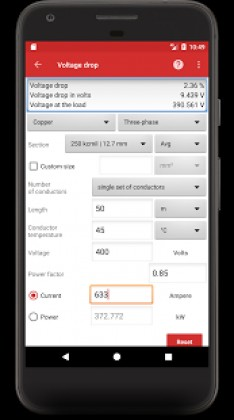# Mobile Electrician Pro 4.3 Apk latest

event_note28 Jan 2019

Mobile Electrician Pro 4.3 Apk Paid Full latest is a Productivity Android app

Mobile Electrician Pro is a Productivity android app made by Anatoliy Tarasenko that you can install on your android devices an enjoy !
Basic electrical calculations on your mobile phone.
The PRO version of Mobile Electrician doesn’t have any ads.

The app includes:

Ohms law for DC.
Ohms law for AC.
L-C Resonance Calculator.
Y-Δ Transformation.
Electrical Energy Calculator.
Current density.
Basic transformer calculations.
Calculate AC circuits
Connection of capacitors.
Capacitive reactance.
Run a 3 phase motor on a single phase.
Capacitive Voltage Divider.
Capacitor Charge / Energy Calculator.
Capacitor alphanumeric markings.
Connection of resistors.
Voltage divider.
LED Resistor.
Decode the color bands resistors.
Encode the color bands resistors.
Wheatstone Bridge Calculator.
Resistor alphanumeric markings.
Conductor Resistance.
Conductor length.
Conductor cross section (by the formula).
Voltage drop.
Electrical resistivity and conductivity.
Inductive reactance cable.
Maximum length of conductor.
Calculation Amps motor.
Calculation power motor.
Calculation kVa (3 Phase) motor.
Calculation Power Factor(3 Phase).
Calculation efficiency.
Calculation of the motor slip.
Calculation motor torque.
Individual compensation three-phase induction motors.
Calculation motor power for centrifugal pump.
Calculation motor power for piston compressor.
Calculation motor power for the fan.
Three-Phase Motor Voltage Unbalance.
Three-Phase Motor Current Unbalance.
Allowable ampacities for wire (PUE – Rules of electrical facilities maintenance).
Wire Size Calculator (PUE).
Motor protection.(PUE).
Maximum circuit conductor length.(PUE).
Maximum circuit ampacity.(PUE).
Allowable ampacities for wire (National Electrical Code).
Wire Size Calculator (NEC).
Conduit fill calculator (NEC).
Maximum circuit conductor length.(NEC).
Maximum circuit ampacity.(NEC).
Allowable ampacities for wire (Canadian Electrical Code).
Wire Size Calculator (CEC).
Maximum circuit conductor length (CEC).
Maximum circuit ampacity (CEC).
Allowable ampacities for wire IEC 60364-5-52 (International Electrotechnical Commission).
Wire Size Calculator (IEC).
Maximum circuit conductor length (IEC).
Maximum circuit ampacity (IEC).
Minimum levels of short-circuit current.
Calculate fuse.
Leakage current.
Heating Element use Nichrome.
Calculation sizing a generator for your home.
Calculation of electrical loads applying demand factor
Calculate number cables that fits in a pipe.
IP Code.
Cable Reel Capacity.
Length of cable on a reel.
Calculate cable conductor weight
Cable weight by formulas
Wiring Color Codes
Length of a Hanging Cable
Power losses in cable
Appliance classes
Short-circuit current at the secondary terminals of a MV/LV distribution transformer
Power of heating element
Force of an Electromagnet
Conversion wire size.
Conversion: Active power – Real power – Reactive power.
Calculation Reactive Power Compensation.
Translations AWG (American Wire gauge) in inches, millimeters, millimeters square and back.
Conversion cos(φ)-sin(φ)-tg(φ).
Conversion of units of temperature.
Conversion of units of distance.
Conversion of units of pressure.
Conversion units of flow.
Conversion units of volume.
Conversion voltage(Peak – RMS-..)
Conversion units of energy.
Conversion SI prefixes.
Converter weight
Time Units Conversion

Electricity Cost Calculator
Print
Mathematical calculator
Percentage calculator
Online and offline handbook (NEC 2011, PUE Ukraine, PUE Russia ,and more..).
Online and Offline Search in the handbook.
Save the result of some calculation in HTML format, and then view and send.
For each calculation is given a separate help.
Dark and light themes application.

If you like the application, please rate it.Mobile Electrician Pro ApkMobile Electrician Pro Apk

Whats New:
Now reports are available for all calculations.
Other modifications and improvements.
Bugs fixed.